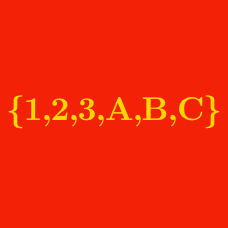Probability

# Sets - Subsets

If $S=\{2,6,8\},$ which of the following is the power set of $S?$

A. $\{ \{2\}, \{6\}, \{8\}, \{2,6\}, \{2,8\},\{6,8\}, \{2,6,8\} \}$

B. $\{ \emptyset, \{2\}, \{6\}, \{8\}, \{2,6\}, \{2,8\},\{6,8\}, \{2,6,8\} \}$

C. $\{ \emptyset, \{2\}, \{6\}, \{8\}, \{2,6\}, \{2,8\},\{6,8\} \}$

D. $\{ \{2\}, \{6\}, \{8\}, \{2,6\}, \{2,8\},\{6,8\} \}$

Let $X=\{6, -\alpha\}, Y=\{\alpha^2+5,\alpha-8,1\}.$ If $X \subseteq Y,$ what is the real number $\alpha?$

Given the set $A=\{x \mid x \text{ is a positive integer } < 13\},$ how many subsets of $A$ have no even numbers?

Details and assumptions

The empty set $\emptyset$ has no elements, and hence, has no even numbers.

If $A=\{5, 6, 7\}$ and $B=\{6, 7, 9, 11, 15\},$ how many sets $X$ are there satisfying

$(A-B) \cup X = X, (A \cup B) \cap X = X?$

If $A$ is the set

$A=\{5, 7, 11, 14\},$ how many subsets of $A$ do not contain the element $7?$

×